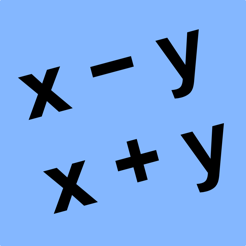## Screenshots

•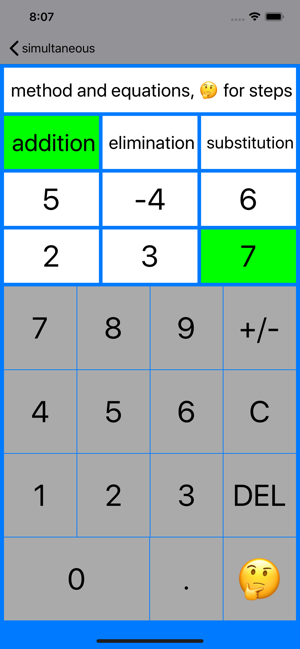•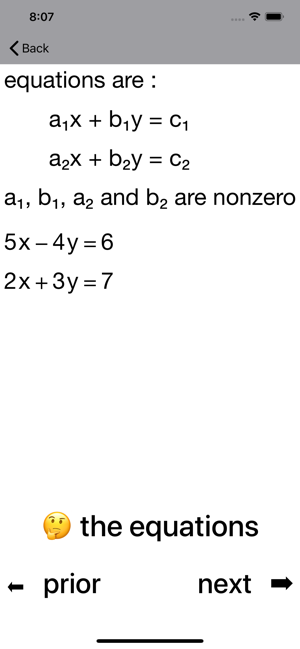•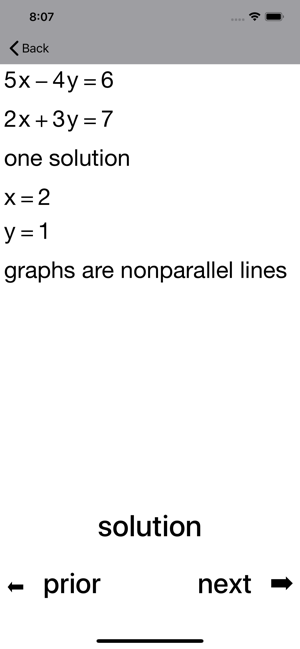•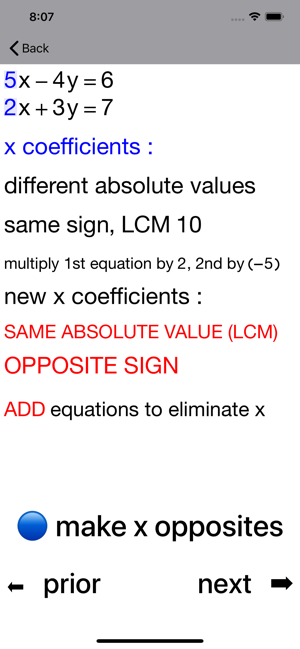•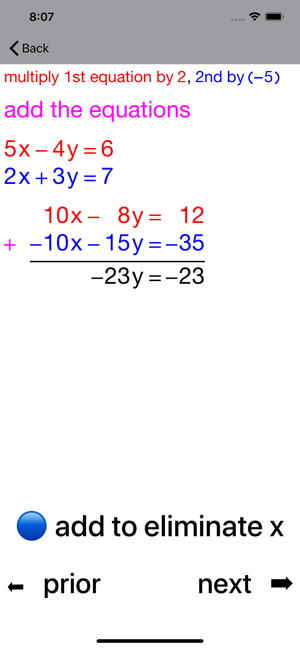•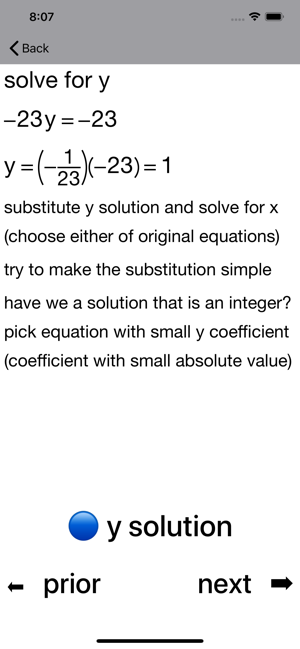•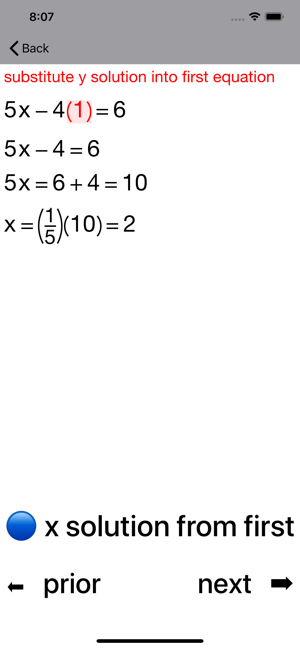•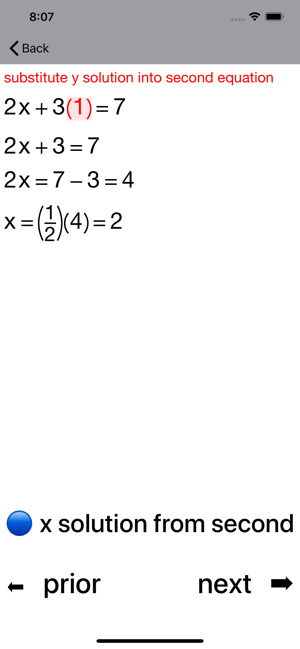## Description

A power learning aid combining Coaching Calculators and Guides to take you step-by-step through the solving of a pair of simultaneous linear equations.

The app features the addition method, the elimination method and the substitution method.

Coefficients can be nonzero integers from -99 to 99 inclusive.

You develop skills quickly as you work with the calculator and see each step of the process in detail :

- Choose which variable to eliminate
- Decide how to make its coefficients opposites
- Manipulate equations to make its coefficients opposites
- Eliminate the variable by adding the equations
- Solve resulting equation for the other variable
- Back substitute into an original equation

Elimination method :

- Choose which variable to eliminate
- Decide how to make its coefficients the same (absolute value)
- Manipulate equations appropriately
- Eliminate the variable by adding/subtracting equations
- Solve resulting equation for the other variable
- Back substitute into an original equation

Substitution method :

The steps if you start by solving first equation to express x in terms of y are shown here.

- Solve first equation to express x in terms of y
- Substitute x in second equation and solve for y
- Substitute y solution back into rearranged first equation
- Solve resulting equation for x

Note that the Coaching Calculator shows the steps if you start by solving x or y from either equation.

Version 4.4

## Information

Seller
Walter Kissach
Size
10.7 MB
Category
Education
Compatibility

Requires iOS 13.0 or later. Compatible with iPhone, iPad, and iPod touch.

Languages

English

Age Rating
4+
Price
\$0.99

## Supports

•